# Basic C Structures and Operations¶

The section describes the main data structures, used by the OpenCV 1.x API, and the basic functions to create and process the data structures.

## CvPoint¶

C: CvPoint cvPoint(int x, int y)

constructs CvPoint structure.

C: CvPoint cvPointFrom32f(CvPoint2D32f point)

converts CvPoint2D32f to CvPoint.

struct CvPoint

2D point with integer coordinates (usually zero-based).

param x: x-coordinate of the point. y-coordinate of the point. the point to convert.

Point_

## CvPoint2D32f¶

C: CvPoint2D32f cvPoint2D32f(double x, double y)

constructs CvPoint2D32f structure.

C: CvPoint2D32f cvPointTo32f(CvPoint point)

converts CvPoint to CvPoint2D32f.

struct CvPoint2D32f

2D point with floating-point coordinates.

param x: floating-point x-coordinate of the point. floating-point y-coordinate of the point. the point to convert.

Point_

## CvPoint3D32f¶

struct CvPoint3D32f

3D point with floating-point coordinates

C: CvPoint3D32f cvPoint3D32f(double x, double y, double z)

constructs CvPoint3D32f structure.

Parameters: x – floating-point x-coordinate of the point. y – floating-point y-coordinate of the point. z – floating-point z-coordinate of the point.

Point3_

## CvPoint2D64f¶

struct CvPoint2D64f

2D point with double-precision floating-point coordinates.

C: CvPoint2D64f cvPoint2D64f(double x, double y)

constructs CvPoint2D64f structure.

Parameters: x – double-precision floating-point x-coordinate of the point. y – double-precision floating-point y-coordinate of the point.

Point_

## CvPoint3D64f¶

struct CvPoint3D64f

3D point with double-precision floating-point coordinates.

C: CvPoint3D64f cvPoint3D64f(double x, double y, double z)

constructs CvPoint3D64f structure.

Parameters: x – double-precision floating-point x-coordinate of the point. y – double-precision floating-point y-coordinate of the point. z – double-precision floating-point z-coordinate of the point.

Point3_

## CvSize¶

struct CvSize

Size of a rectangle or an image.

C: CvSize cvSize(int width, int height)

constructs CvSize structure.

Parameters: width – width of the rectangle. height – height of the rectangle.

Size_

## CvSize2D32f¶

struct CvSize2D32f

Sub-pixel accurate size of a rectangle.

C: CvSize2D32f cvSize2D32f(double width, double height)

constructs CvSize2D32f structure.

Parameters: width – floating-point width of the rectangle. height – floating-point height of the rectangle.

Size_

## CvRect¶

struct CvRect

Stores coordinates of a rectangle.

C: CvRect cvRect(int x, int y, int width, int height)

constructs CvRect structure.

Parameters: x – x-coordinate of the top-left corner. y – y-coordinate of the top-left corner (sometimes bottom-left corner). width – width of the rectangle. height – height of the rectangle.

Rect_

## CvBox2D¶

struct CvBox2D

Stores coordinates of a rotated rectangle.

CvPoint2D32f center

Center of the box

CvSize2D32f size

Box width and height

float angle

Angle between the horizontal axis and the first side (i.e. length) in degrees

RotatedRect

## CvScalar¶

struct CvScalar

A container for 1-,2-,3- or 4-tuples of doubles.

double val

Scalar_

## CvTermCriteria¶

struct CvTermCriteria

Termination criteria for iterative algorithms.

int type

type of the termination criteria, one of:

• CV_TERMCRIT_ITER - stop the algorithm after max_iter iterations at maximum.
• CV_TERMCRIT_EPS - stop the algorithm after the achieved algorithm-dependent accuracy becomes lower than epsilon.
• CV_TERMCRIT_ITER+CV_TERMCRIT_EPS - stop the algorithm after max_iter iterations or when the achieved accuracy is lower than epsilon, whichever comes the earliest.
int max_iter

Maximum number of iterations

double epsilon

Required accuracy

TermCriteria

## CvMat¶

struct CvMat

A multi-channel dense matrix.

int type

CvMat signature (CV_MAT_MAGIC_VAL) plus type of the elements. Type of the matrix elements can be retrieved using CV_MAT_TYPE macro:

int type = CV_MAT_TYPE(matrix->type);


For description of possible matrix elements, see Mat.

int step

Full row length in bytes

int* refcount

Underlying data reference counter

union data

Pointers to the actual matrix data:

• ptr - pointer to 8-bit unsigned elements
• s - pointer to 16-bit signed elements
• i - pointer to 32-bit signed elements
• fl - pointer to 32-bit floating-point elements
• db - pointer to 64-bit floating-point elements
int rows

Number of rows

int cols

Number of columns

Matrix elements are stored row by row. Element (i, j) (i - 0-based row index, j - 0-based column index) of a matrix can be retrieved or modified using CV_MAT_ELEM macro:

uchar pixval = CV_MAT_ELEM(grayimg, uchar, i, j)
CV_MAT_ELEM(cameraMatrix, float, 0, 2) = image.width*0.5f;


To access multiple-channel matrices, you can use CV_MAT_ELEM(matrix, type, i, j*nchannels + channel_idx).

CvMat is now obsolete; consider using Mat instead.

## CvMatND¶

struct CvMatND

Multi-dimensional dense multi-channel array.

int type

A CvMatND signature (CV_MATND_MAGIC_VAL) plus the type of elements. Type of the matrix elements can be retrieved using CV_MAT_TYPE macro:

int type = CV_MAT_TYPE(ndmatrix->type);

int dims

The number of array dimensions

int* refcount

Underlying data reference counter

union data

Pointers to the actual matrix data

• ptr - pointer to 8-bit unsigned elements
• s - pointer to 16-bit signed elements
• i - pointer to 32-bit signed elements
• fl - pointer to 32-bit floating-point elements
• db - pointer to 64-bit floating-point elements
array dim

Arrays of pairs (array size along the i-th dimension, distance between neighbor elements along i-th dimension):

for(int i = 0; i < ndmatrix->dims; i++)
printf("size[i] = %d, step[i] = %d\n", ndmatrix->dim[i].size, ndmatrix->dim[i].step);


CvMatND is now obsolete; consider using Mat instead.

## CvSparseMat¶

struct CvSparseMat

Multi-dimensional sparse multi-channel array.

int type

A CvSparseMat signature (CV_SPARSE_MAT_MAGIC_VAL) plus the type of sparse matrix elements. Similarly to CvMat and CvMatND, use CV_MAT_TYPE() to retrieve type of the elements.

int dims

Number of dimensions

int* refcount

Underlying reference counter. Not used.

CvSet* heap

A pool of hash table nodes

void** hashtable

The hash table. Each entry is a list of nodes.

int hashsize

Size of the hash table

int[] size

Array of dimension sizes

## IplImage¶

struct IplImage

int nSize

sizeof(IplImage)

int ID

Version, always equals 0

int nChannels

Number of channels. Most OpenCV functions support 1-4 channels.

int alphaChannel

Ignored by OpenCV

int depth

Channel depth in bits + the optional sign bit ( IPL_DEPTH_SIGN ). The supported depths are:

• IPL_DEPTH_8U - unsigned 8-bit integer. Equivalent to CV_8U in matrix types.
• IPL_DEPTH_8S - signed 8-bit integer. Equivalent to CV_8S in matrix types.
• IPL_DEPTH_16U - unsigned 16-bit integer. Equivalent to CV_16U in matrix types.
• IPL_DEPTH_16S - signed 8-bit integer. Equivalent to CV_16S in matrix types.
• IPL_DEPTH_32S - signed 32-bit integer. Equivalent to CV_32S in matrix types.
• IPL_DEPTH_32F - single-precision floating-point number. Equivalent to CV_32F in matrix types.
• IPL_DEPTH_64F - double-precision floating-point number. Equivalent to CV_64F in matrix types.
char[] colorModel

Ignored by OpenCV.

char[] channelSeq

Ignored by OpenCV

int dataOrder

0 = IPL_DATA_ORDER_PIXEL - interleaved color channels, 1 - separate color channels. CreateImage() only creates images with interleaved channels. For example, the usual layout of a color image is: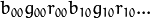int origin

0 - top-left origin, 1 - bottom-left origin (Windows bitmap style)

int align

Alignment of image rows (4 or 8). OpenCV ignores this and uses widthStep instead.

int width

Image width in pixels

int height

Image height in pixels

IplROI* roi

Region Of Interest (ROI). If not NULL, only this image region will be processed.

Must be NULL in OpenCV

void* imageId

Must be NULL in OpenCV

void* tileInfo

Must be NULL in OpenCV

int imageSize

Image data size in bytes. For interleaved data, this equals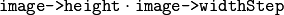char* imageData

A pointer to the aligned image data. Do not assign imageData directly. Use SetData().

int widthStep

The size of an aligned image row, in bytes.

int[] BorderMode

Border completion mode, ignored by OpenCV

int[] BorderConst

Constant border value, ignored by OpenCV

char* imageDataOrigin

A pointer to the origin of the image data (not necessarily aligned). This is used for image deallocation.

The IplImage is taken from the Intel Image Processing Library, in which the format is native. OpenCV only supports a subset of possible IplImage formats, as outlined in the parameter list above.

In addition to the above restrictions, OpenCV handles ROIs differently. OpenCV functions require that the image size or ROI size of all source and destination images match exactly. On the other hand, the Intel Image Processing Library processes the area of intersection between the source and destination images (or ROIs), allowing them to vary independently.

## CvArr¶

struct CvArr

This is the “metatype” used only as a function parameter. It denotes that the function accepts arrays of multiple types, such as IplImage*, CvMat* or even CvSeq* sometimes. The particular array type is determined at runtime by analyzing the first 4 bytes of the header. In C++ interface the role of CvArr is played by InputArray and OutputArray.

## ClearND¶

Clears a specific array element.

C: void cvClearND(CvArr* arr, const int* idx)
Parameters: arr – Input array idx – Array of the element indices

The function clears (sets to zero) a specific element of a dense array or deletes the element of a sparse array. If the sparse array element does not exists, the function does nothing.

## CloneImage¶

Makes a full copy of an image, including the header, data, and ROI.

C: IplImage* cvCloneImage(const IplImage* image)
Parameters: image – The original image

## CloneMat¶

Creates a full matrix copy.

C: CvMat* cvCloneMat(const CvMat* mat)
Parameters: mat – Matrix to be copied

Creates a full copy of a matrix and returns a pointer to the copy. Note that the matrix copy is compacted, that is, it will not have gaps between rows.

## CloneMatND¶

Creates full copy of a multi-dimensional array and returns a pointer to the copy.

C: CvMatND* cvCloneMatND(const CvMatND* mat)
Parameters: mat – Input array

## CloneSparseMat¶

Creates full copy of sparse array.

C: CvSparseMat* cvCloneSparseMat(const CvSparseMat* mat)
Parameters: mat – Input array

The function creates a copy of the input array and returns pointer to the copy.

## ConvertScale¶

Converts one array to another with optional linear transformation.

C: void cvConvertScale(const CvArr* src, CvArr* dst, double scale=1, double shift=0)
#define cvCvtScale cvConvertScale
#define cvScale  cvConvertScale
#define cvConvert(src, dst )  cvConvertScale((src), (dst), 1, 0 )

Parameters: src – Source array dst – Destination array scale – Scale factor shift – Value added to the scaled source array elements

The function has several different purposes, and thus has several different names. It copies one array to another with optional scaling, which is performed first, and/or optional type conversion, performed after: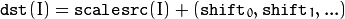All the channels of multi-channel arrays are processed independently.

The type of conversion is done with rounding and saturation, that is if the result of scaling + conversion can not be represented exactly by a value of the destination array element type, it is set to the nearest representable value on the real axis.

## Copy¶

Copies one array to another.

C: void cvCopy(const CvArr* src, CvArr* dst, const CvArr* mask=NULL)
Parameters: src – The source array dst – The destination array mask – Operation mask, 8-bit single channel array; specifies elements of the destination array to be changed

The function copies selected elements from an input array to an output array: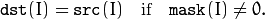If any of the passed arrays is of IplImage type, then its ROI and COI fields are used. Both arrays must have the same type, the same number of dimensions, and the same size. The function can also copy sparse arrays (mask is not supported in this case).

## CreateData¶

Allocates array data

C: void cvCreateData(CvArr* arr)

The function allocates image, matrix or multi-dimensional dense array data. Note that in the case of matrix types OpenCV allocation functions are used. In the case of IplImage they are used unless CV_TURN_ON_IPL_COMPATIBILITY() has been called before. In the latter case IPL functions are used to allocate the data.

## CreateImage¶

Creates an image header and allocates the image data.

C: IplImage* cvCreateImage(CvSize size, int depth, int channels)
Parameters: size – Image width and height depth – Bit depth of image elements. See IplImage for valid depths. channels – Number of channels per pixel. See IplImage for details. This function only creates images with interleaved channels.

This function call is equivalent to the following code:

header = cvCreateImageHeader(size, depth, channels);


Creates an image header but does not allocate the image data.

C: IplImage* cvCreateImageHeader(CvSize size, int depth, int channels)
Parameters: size – Image width and height depth – Image depth (see CreateImage() ) channels – Number of channels (see CreateImage() )

## CreateMat¶

Creates a matrix header and allocates the matrix data.

C: CvMat* cvCreateMat(int rows, int cols, int type)
Parameters: rows – Number of rows in the matrix cols – Number of columns in the matrix type – The type of the matrix elements in the form CV_C , where S=signed, U=unsigned, F=float. For example, CV _ 8UC1 means the elements are 8-bit unsigned and the there is 1 channel, and CV _ 32SC2 means the elements are 32-bit signed and there are 2 channels.

The function call is equivalent to the following code:

CvMat* mat = cvCreateMatHeader(rows, cols, type);
cvCreateData(mat);


Creates a matrix header but does not allocate the matrix data.

C: CvMat* cvCreateMatHeader(int rows, int cols, int type)
Parameters: rows – Number of rows in the matrix cols – Number of columns in the matrix type – Type of the matrix elements, see CreateMat()

The function allocates a new matrix header and returns a pointer to it. The matrix data can then be allocated using CreateData() or set explicitly to user-allocated data via SetData().

## CreateMatND¶

Creates the header and allocates the data for a multi-dimensional dense array.

C: CvMatND* cvCreateMatND(int dims, const int* sizes, int type)
Parameters: dims – Number of array dimensions. This must not exceed CV_MAX_DIM (32 by default, but can be changed at build time). sizes – Array of dimension sizes. type – Type of array elements, see CreateMat() .

This function call is equivalent to the following code:

CvMatND* mat = cvCreateMatNDHeader(dims, sizes, type);
cvCreateData(mat);


Creates a new matrix header but does not allocate the matrix data.

C: CvMatND* cvCreateMatNDHeader(int dims, const int* sizes, int type)
Parameters: dims – Number of array dimensions sizes – Array of dimension sizes type – Type of array elements, see CreateMat()

The function allocates a header for a multi-dimensional dense array. The array data can further be allocated using CreateData() or set explicitly to user-allocated data via SetData().

## CreateSparseMat¶

Creates sparse array.

C: CvSparseMat* cvCreateSparseMat(int dims, const int* sizes, int type)
Parameters: dims – Number of array dimensions. In contrast to the dense matrix, the number of dimensions is practically unlimited (up to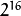). sizes – Array of dimension sizes type – Type of array elements. The same as for CvMat

The function allocates a multi-dimensional sparse array. Initially the array contain no elements, that is PtrND() and other related functions will return 0 for every index.

## CrossProduct¶

Calculates the cross product of two 3D vectors.

C: void cvCrossProduct(const CvArr* src1, const CvArr* src2, CvArr* dst)
Parameters: src1 – The first source vector src2 – The second source vector dst – The destination vector

The function calculates the cross product of two 3D vectors: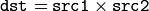or: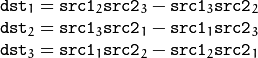## DotProduct¶

Calculates the dot product of two arrays in Euclidean metrics.

C: double cvDotProduct(const CvArr* src1, const CvArr* src2)
Parameters: src1 – The first source array src2 – The second source array

The function calculates and returns the Euclidean dot product of two arrays.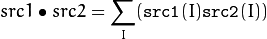In the case of multiple channel arrays, the results for all channels are accumulated. In particular, cvDotProduct(a,a) where a is a complex vector, will return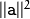. The function can process multi-dimensional arrays, row by row, layer by layer, and so on.

## Get?D¶

C: CvScalar cvGet1D(const CvArr* arr, int idx0)
C: CvScalar cvGet2D(const CvArr* arr, int idx0, int idx1)
C: CvScalar cvGet3D(const CvArr* arr, int idx0, int idx1, int idx2)
C: CvScalar cvGetND(const CvArr* arr, const int* idx)

Return a specific array element.

Parameters: arr – Input array idx0 – The first zero-based component of the element index idx1 – The second zero-based component of the element index idx2 – The third zero-based component of the element index idx – Array of the element indices

The functions return a specific array element. In the case of a sparse array the functions return 0 if the requested node does not exist (no new node is created by the functions).

## GetCol(s)¶

Returns one of more array columns.

C: CvMat* cvGetCol(const CvArr* arr, CvMat* submat, int col)
C: CvMat* cvGetCols(const CvArr* arr, CvMat* submat, int start_col, int end_col)
Parameters: arr – Input array submat – Pointer to the resulting sub-array header col – Zero-based index of the selected column start_col – Zero-based index of the starting column (inclusive) of the span end_col – Zero-based index of the ending column (exclusive) of the span

The functions return the header, corresponding to a specified column span of the input array. That is, no data is copied. Therefore, any modifications of the submatrix will affect the original array. If you need to copy the columns, use CloneMat(). cvGetCol(arr, submat, col) is a shortcut for cvGetCols(arr, submat, col, col+1).

## GetDiag¶

Returns one of array diagonals.

C: CvMat* cvGetDiag(const CvArr* arr, CvMat* submat, int diag=0)
Parameters: arr – Input array submat – Pointer to the resulting sub-array header diag – Index of the array diagonal. Zero value corresponds to the main diagonal, -1 corresponds to the diagonal above the main, 1 corresponds to the diagonal below the main, and so forth.

The function returns the header, corresponding to a specified diagonal of the input array.

## GetDims¶

Return number of array dimensions

C: int cvGetDims(const CvArr* arr, int* sizes=NULL)
Parameters: arr – Input array sizes – Optional output vector of the array dimension sizes. For 2d arrays the number of rows (height) goes first, number of columns (width) next.

The function returns the array dimensionality and the array of dimension sizes. In the case of IplImage or CvMat it always returns 2 regardless of number of image/matrix rows. For example, the following code calculates total number of array elements:

int sizes[CV_MAX_DIM];
int i, total = 1;
int dims = cvGetDims(arr, size);
for(i = 0; i < dims; i++ )
total *= sizes[i];


## GetDimSize¶

Returns array size along the specified dimension.

C: int cvGetDimSize(const CvArr* arr, int index)
Parameters: arr – Input array index – Zero-based dimension index (for matrices 0 means number of rows, 1 means number of columns; for images 0 means height, 1 means width)

## GetElemType¶

Returns type of array elements.

C: int cvGetElemType(const CvArr* arr)
Parameters: arr – Input array

The function returns type of the array elements. In the case of IplImage the type is converted to CvMat-like representation. For example, if the image has been created as:

IplImage* img = cvCreateImage(cvSize(640, 480), IPL_DEPTH_8U, 3);


The code cvGetElemType(img) will return CV_8UC3.

## GetImage¶

Returns image header for arbitrary array.

C: IplImage* cvGetImage(const CvArr* arr, IplImage* image_header)
Parameters: arr – Input array image_header – Pointer to IplImage structure used as a temporary buffer

The function returns the image header for the input array that can be a matrix (CvMat) or image (IplImage). In the case of an image the function simply returns the input pointer. In the case of CvMat it initializes an image_header structure with the parameters of the input matrix. Note that if we transform IplImage to CvMat using GetMat() and then transform CvMat back to IplImage using this function, we will get different headers if the ROI is set in the original image.

## GetImageCOI¶

Returns the index of the channel of interest.

C: int cvGetImageCOI(const IplImage* image)
Parameters: image – A pointer to the image header

Returns the channel of interest of in an IplImage. Returned values correspond to the coi in SetImageCOI().

## GetImageROI¶

Returns the image ROI.

C: CvRect cvGetImageROI(const IplImage* image)
Parameters: image – A pointer to the image header

If there is no ROI set, cvRect(0,0,image->width,image->height) is returned.

## GetMat¶

Returns matrix header for arbitrary array.

C: CvMat* cvGetMat(const CvArr* arr, CvMat* header, int* coi=NULL, int allowND=0)
Parameters: arr – Input array header – Pointer to CvMat structure used as a temporary buffer coi – Optional output parameter for storing COI allowND – If non-zero, the function accepts multi-dimensional dense arrays (CvMatND*) and returns 2D matrix (if CvMatND has two dimensions) or 1D matrix (when CvMatND has 1 dimension or more than 2 dimensions). The CvMatND array must be continuous.

The function returns a matrix header for the input array that can be a matrix - CvMat, an image - IplImage, or a multi-dimensional dense array - CvMatND (the third option is allowed only if allowND != 0) . In the case of matrix the function simply returns the input pointer. In the case of IplImage* or CvMatND it initializes the header structure with parameters of the current image ROI and returns &header. Because COI is not supported by CvMat, it is returned separately.

The function provides an easy way to handle both types of arrays - IplImage and CvMat using the same code. Input array must have non-zero data pointer, otherwise the function will report an error.

Note

If the input array is IplImage with planar data layout and COI set, the function returns the pointer to the selected plane and COI == 0. This feature allows user to process IplImage structures with planar data layout, even though OpenCV does not support such images.

## GetNextSparseNode¶

Returns the next sparse matrix element

C: CvSparseNode* cvGetNextSparseNode(CvSparseMatIterator* mat_iterator)
Parameters: mat_iterator – Sparse array iterator

The function moves iterator to the next sparse matrix element and returns pointer to it. In the current version there is no any particular order of the elements, because they are stored in the hash table. The sample below demonstrates how to iterate through the sparse matrix:

// print all the non-zero sparse matrix elements and compute their sum
double sum = 0;
int i, dims = cvGetDims(sparsemat);
CvSparseMatIterator it;
CvSparseNode* node = cvInitSparseMatIterator(sparsemat, &it);

for(; node != 0; node = cvGetNextSparseNode(&it))
{
/* get pointer to the element indices */
int* idx = CV_NODE_IDX(array, node);
/* get value of the element (assume that the type is CV_32FC1) */
float val = *(float*)CV_NODE_VAL(array, node);
printf("M");
for(i = 0; i < dims; i++ )
printf("[%d]", idx[i]);
printf("=%g\n", val);

sum += val;
}

printf("nTotal sum = %g\n", sum);


## GetRawData¶

Retrieves low-level information about the array.

C: void cvGetRawData(const CvArr* arr, uchar** data, int* step=NULL, CvSize* roi_size=NULL )
Parameters: arr – Array header data – Output pointer to the whole image origin or ROI origin if ROI is set step – Output full row length in bytes roi_size – Output ROI size

The function fills output variables with low-level information about the array data. All output parameters are optional, so some of the pointers may be set to NULL. If the array is IplImage with ROI set, the parameters of ROI are returned.

The following example shows how to get access to array elements. It computes absolute values of the array elements

float* data;
int step;
CvSize size;

cvGetRawData(array, (uchar**)&data, &step, &size);
step /= sizeof(data);

for(int y = 0; y < size.height; y++, data += step )
for(int x = 0; x < size.width; x++ )
data[x] = (float)fabs(data[x]);


## GetReal?D¶

Return a specific element of single-channel 1D, 2D, 3D or nD array.

C: double cvGetReal1D(const CvArr* arr, int idx0)
C: double cvGetReal2D(const CvArr* arr, int idx0, int idx1)
C: double cvGetReal3D(const CvArr* arr, int idx0, int idx1, int idx2)
C: double cvGetRealND(const CvArr* arr, const int* idx)
Parameters: arr – Input array. Must have a single channel. idx0 – The first zero-based component of the element index idx1 – The second zero-based component of the element index idx2 – The third zero-based component of the element index idx – Array of the element indices

Returns a specific element of a single-channel array. If the array has multiple channels, a runtime error is raised. Note that Get?D functions can be used safely for both single-channel and multiple-channel arrays though they are a bit slower.

In the case of a sparse array the functions return 0 if the requested node does not exist (no new node is created by the functions).

## GetRow(s)¶

Returns array row or row span.

C: CvMat* cvGetRow(const CvArr* arr, CvMat* submat, int row)
C: CvMat* cvGetRows(const CvArr* arr, CvMat* submat, int start_row, int end_row, int delta_row=1 )
Parameters: arr – Input array submat – Pointer to the resulting sub-array header row – Zero-based index of the selected row start_row – Zero-based index of the starting row (inclusive) of the span end_row – Zero-based index of the ending row (exclusive) of the span delta_row – Index step in the row span. That is, the function extracts every delta_row -th row from start_row and up to (but not including) end_row .

The functions return the header, corresponding to a specified row/row span of the input array. cvGetRow(arr, submat, row) is a shortcut for cvGetRows(arr, submat, row, row+1).

## GetSize¶

Returns size of matrix or image ROI.

C: CvSize cvGetSize(const CvArr* arr)

The function returns number of rows (CvSize::height) and number of columns (CvSize::width) of the input matrix or image. In the case of image the size of ROI is returned.

## GetSubRect¶

Returns matrix header corresponding to the rectangular sub-array of input image or matrix.

C: CvMat* cvGetSubRect(const CvArr* arr, CvMat* submat, CvRect rect)
Parameters: arr – Input array submat – Pointer to the resultant sub-array header rect – Zero-based coordinates of the rectangle of interest

The function returns header, corresponding to a specified rectangle of the input array. In other words, it allows the user to treat a rectangular part of input array as a stand-alone array. ROI is taken into account by the function so the sub-array of ROI is actually extracted.

## DecRefData¶

Decrements an array data reference counter.

C: void cvDecRefData(CvArr* arr)
Parameters: arr – Pointer to an array header

The function decrements the data reference counter in a CvMat or CvMatND if the reference counter pointer is not NULL. If the counter reaches zero, the data is deallocated. In the current implementation the reference counter is not NULL only if the data was allocated using the CreateData() function. The counter will be NULL in other cases such as: external data was assigned to the header using SetData(), header is part of a larger matrix or image, or the header was converted from an image or n-dimensional matrix header.

## IncRefData¶

Increments array data reference counter.

C: int cvIncRefData(CvArr* arr)

The function increments CvMat or CvMatND data reference counter and returns the new counter value if the reference counter pointer is not NULL, otherwise it returns zero.

Initializes an image header that was previously allocated.

C: IplImage* cvInitImageHeader(IplImage* image, CvSize size, int depth, int channels, int origin=0, int align=4)
Parameters: image – Image header to initialize size – Image width and height depth – Image depth (see CreateImage() ) channels – Number of channels (see CreateImage() ) origin – Top-left IPL_ORIGIN_TL or bottom-left IPL_ORIGIN_BL align – Alignment for image rows, typically 4 or 8 bytes

The returned IplImage* points to the initialized header.

C: CvMat* cvInitMatHeader(CvMat* mat, int rows, int cols, int type, void* data=NULL, int step=CV_AUTOSTEP)
Parameters: mat – A pointer to the matrix header to be initialized rows – Number of rows in the matrix cols – Number of columns in the matrix type – Type of the matrix elements, see CreateMat() . data – Optional: data pointer assigned to the matrix header step – Optional: full row width in bytes of the assigned data. By default, the minimal possible step is used which assumes there are no gaps between subsequent rows of the matrix.

This function is often used to process raw data with OpenCV matrix functions. For example, the following code computes the matrix product of two matrices, stored as ordinary arrays:

double a[] = { 1, 2, 3, 4,
5, 6, 7, 8,
9, 10, 11, 12 };

double b[] = { 1, 5, 9,
2, 6, 10,
3, 7, 11,
4, 8, 12 };

double c;
CvMat Ma, Mb, Mc ;

// the c array now contains the product of a (3x4) and b (4x3)


Initializes a pre-allocated multi-dimensional array header.

C: CvMatND* cvInitMatNDHeader(CvMatND* mat, int dims, const int* sizes, int type, void* data=NULL)
Parameters: mat – A pointer to the array header to be initialized dims – The number of array dimensions sizes – An array of dimension sizes type – Type of array elements, see CreateMat() data – Optional data pointer assigned to the matrix header

## InitSparseMatIterator¶

Initializes sparse array elements iterator.

C: CvSparseNode* cvInitSparseMatIterator(const CvSparseMat* mat, CvSparseMatIterator* mat_iterator)
Parameters: mat – Input array mat_iterator – Initialized iterator

The function initializes iterator of sparse array elements and returns pointer to the first element, or NULL if the array is empty.

## Mat¶

C: CvMat cvMat(int rows, int cols, int type, void* data=NULL)
Parameters: rows – Number of rows in the matrix cols – Number of columns in the matrix type – Type of the matrix elements - see CreateMat() data – Optional data pointer assigned to the matrix header

Initializes a matrix header and assigns data to it. The matrix is filled row-wise (the first cols elements of data form the first row of the matrix, etc.)

This function is a fast inline substitution for InitMatHeader(). Namely, it is equivalent to:

CvMat mat;
cvInitMatHeader(&mat, rows, cols, type, data, CV_AUTOSTEP);


## Ptr?D¶

Return pointer to a particular array element.

C: uchar* cvPtr1D(const CvArr* arr, int idx0, int* type=NULL)
C: uchar* cvPtr2D(const CvArr* arr, int idx0, int idx1, int* type=NULL)
C: uchar* cvPtr3D(const CvArr* arr, int idx0, int idx1, int idx2, int* type=NULL)
C: uchar* cvPtrND(const CvArr* arr, const int* idx, int* type=NULL, int create_node=1, unsigned int* precalc_hashval=NULL )
Parameters: arr – Input array idx0 – The first zero-based component of the element index idx1 – The second zero-based component of the element index idx2 – The third zero-based component of the element index idx – Array of the element indices type – Optional output parameter: type of matrix elements create_node – Optional input parameter for sparse matrices. Non-zero value of the parameter means that the requested element is created if it does not exist already. precalc_hashval – Optional input parameter for sparse matrices. If the pointer is not NULL, the function does not recalculate the node hash value, but takes it from the specified location. It is useful for speeding up pair-wise operations (TODO: provide an example)

The functions return a pointer to a specific array element. Number of array dimension should match to the number of indices passed to the function except for cvPtr1D function that can be used for sequential access to 1D, 2D or nD dense arrays.

The functions can be used for sparse arrays as well - if the requested node does not exist they create it and set it to zero.

All these as well as other functions accessing array elements ( GetND() , GetRealND() , Set() , SetND() , SetRealND() ) raise an error in case if the element index is out of range.

## ReleaseData¶

Releases array data.

C: void cvReleaseData(CvArr* arr)

The function releases the array data. In the case of CvMat or CvMatND it simply calls cvDecRefData(), that is the function can not deallocate external data. See also the note to CreateData() .

## ReleaseImage¶

Deallocates the image header and the image data.

C: void cvReleaseImage(IplImage** image)
Parameters: image – Double pointer to the image header

This call is a shortened form of

if(*image )
{
cvReleaseData(*image);
}


Parameters: image – Double pointer to the image header

This call is an analogue of

if(image )
{
*image = 0;
}


but it does not use IPL functions by default (see the CV_TURN_ON_IPL_COMPATIBILITY macro).

## ReleaseMat¶

Deallocates a matrix.

C: void cvReleaseMat(CvMat** mat)
Parameters: mat – Double pointer to the matrix

The function decrements the matrix data reference counter and deallocates matrix header. If the data reference counter is 0, it also deallocates the data.

if(*mat )
cvDecRefData(*mat);
cvFree((void**)mat);


## ReleaseMatND¶

Deallocates a multi-dimensional array.

C: void cvReleaseMatND(CvMatND** mat)
Parameters: mat – Double pointer to the array

The function decrements the array data reference counter and releases the array header. If the reference counter reaches 0, it also deallocates the data.

if(*mat )
cvDecRefData(*mat);
cvFree((void**)mat);


## ReleaseSparseMat¶

Deallocates sparse array.

C: void cvReleaseSparseMat(CvSparseMat** mat)
Parameters: mat – Double pointer to the array

The function releases the sparse array and clears the array pointer upon exit.

## ResetImageROI¶

Resets the image ROI to include the entire image and releases the ROI structure.

C: void cvResetImageROI(IplImage* image)
Parameters: image – A pointer to the image header

This produces a similar result to the following, but in addition it releases the ROI structure.

cvSetImageROI(image, cvRect(0, 0, image->width, image->height ));
cvSetImageCOI(image, 0);


## Reshape¶

Changes shape of matrix/image without copying data.

C: CvMat* cvReshape(const CvArr* arr, CvMat* header, int new_cn, int new_rows=0 )
Parameters: arr – Input array header – Output header to be filled new_cn – New number of channels. ‘new_cn = 0’ means that the number of channels remains unchanged. new_rows – New number of rows. ‘new_rows = 0’ means that the number of rows remains unchanged unless it needs to be changed according to new_cn value.

The function initializes the CvMat header so that it points to the same data as the original array but has a different shape - different number of channels, different number of rows, or both.

The following example code creates one image buffer and two image headers, the first is for a 320x240x3 image and the second is for a 960x240x1 image:

IplImage* color_img = cvCreateImage(cvSize(320,240), IPL_DEPTH_8U, 3);
CvMat gray_mat_hdr;
IplImage gray_img_hdr, *gray_img;
cvReshape(color_img, &gray_mat_hdr, 1);
gray_img = cvGetImage(&gray_mat_hdr, &gray_img_hdr);


And the next example converts a 3x3 matrix to a single 1x9 vector:

CvMat* mat = cvCreateMat(3, 3, CV_32F);
row = cvReshape(mat, &row_header, 0, 1);


## ReshapeMatND¶

Changes the shape of a multi-dimensional array without copying the data.

C: CvArr* cvReshapeMatND(const CvArr* arr, int sizeof_header, CvArr* header, int new_cn, int new_dims, int* new_sizes)
Parameters: arr – Input array sizeof_header – Size of output header to distinguish between IplImage, CvMat and CvMatND output headers header – Output header to be filled new_cn – New number of channels. new_cn = 0 means that the number of channels remains unchanged. new_dims – New number of dimensions. new_dims = 0 means that the number of dimensions remains the same. new_sizes – Array of new dimension sizes. Only new_dims-1 values are used, because the total number of elements must remain the same. Thus, if new_dims = 1, new_sizes array is not used.

The function is an advanced version of Reshape() that can work with multi-dimensional arrays as well (though it can work with ordinary images and matrices) and change the number of dimensions.

Below are the two samples from the Reshape() description rewritten using ReshapeMatND() :

IplImage* color_img = cvCreateImage(cvSize(320,240), IPL_DEPTH_8U, 3);
IplImage gray_img_hdr, *gray_img;
gray_img = (IplImage*)cvReshapeMatND(color_img, sizeof(gray_img_hdr), &gray_img_hdr, 1, 0, 0);

...

/* second example is modified to convert 2x2x2 array to 8x1 vector */
int size[] = { 2, 2, 2 };
CvMatND* mat = cvCreateMatND(3, size, CV_32F);


In C, the header file for this function includes a convenient macro cvReshapeND that does away with the sizeof_header parameter. So, the lines containing the call to cvReshapeMatND in the examples may be replaced as follow:

gray_img = (IplImage*)cvReshapeND(color_img, &gray_img_hdr, 1, 0, 0);

...

row = (CvMat*)cvReshapeND(mat, &row_header, 0, 1, 0);


## Set¶

Sets every element of an array to a given value.

C: void cvSet(CvArr* arr, CvScalar value, const CvArr* mask=NULL)
Parameters: arr – The destination array value – Fill value mask – Operation mask, 8-bit single channel array; specifies elements of the destination array to be changed

The function copies the scalar value to every selected element of the destination array: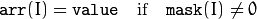If array arr is of IplImage type, then is ROI used, but COI must not be set.

## Set?D¶

Change the particular array element.

C: void cvSet1D(CvArr* arr, int idx0, CvScalar value)
C: void cvSet2D(CvArr* arr, int idx0, int idx1, CvScalar value)
C: void cvSet3D(CvArr* arr, int idx0, int idx1, int idx2, CvScalar value)
C: void cvSetND(CvArr* arr, const int* idx, CvScalar value)
Parameters: arr – Input array idx0 – The first zero-based component of the element index idx1 – The second zero-based component of the element index idx2 – The third zero-based component of the element index idx – Array of the element indices value – The assigned value

The functions assign the new value to a particular array element. In the case of a sparse array the functions create the node if it does not exist yet.

## SetData¶

Assigns user data to the array header.

C: void cvSetData(CvArr* arr, void* data, int step)
Parameters: arr – Array header data – User data step – Full row length in bytes

## SetImageCOI¶

Sets the channel of interest in an IplImage.

C: void cvSetImageCOI(IplImage* image, int coi)
Parameters: image – A pointer to the image header coi – The channel of interest. 0 - all channels are selected, 1 - first channel is selected, etc. Note that the channel indices become 1-based.

If the ROI is set to NULL and the coi is not 0, the ROI is allocated. Most OpenCV functions do not support the COI setting, so to process an individual image/matrix channel one may copy (via Copy() or Split()) the channel to a separate image/matrix, process it and then copy the result back (via Copy() or Merge()) if needed.

## SetImageROI¶

Sets an image Region Of Interest (ROI) for a given rectangle.

C: void cvSetImageROI(IplImage* image, CvRect rect)
Parameters: image – A pointer to the image header rect – The ROI rectangle

If the original image ROI was NULL and the rect is not the whole image, the ROI structure is allocated.

Most OpenCV functions support the use of ROI and treat the image rectangle as a separate image. For example, all of the pixel coordinates are counted from the top-left (or bottom-left) corner of the ROI, not the original image.

## SetReal?D¶

Change a specific array element.

C: void cvSetReal1D(CvArr* arr, int idx0, double value)
C: void cvSetReal2D(CvArr* arr, int idx0, int idx1, double value)
C: void cvSetReal3D(CvArr* arr, int idx0, int idx1, int idx2, double value)
C: void cvSetRealND(CvArr* arr, const int* idx, double value)
Parameters: arr – Input array idx0 – The first zero-based component of the element index idx1 – The second zero-based component of the element index idx2 – The third zero-based component of the element index idx – Array of the element indices value – The assigned value

The functions assign a new value to a specific element of a single-channel array. If the array has multiple channels, a runtime error is raised. Note that the Set*D function can be used safely for both single-channel and multiple-channel arrays, though they are a bit slower.

In the case of a sparse array the functions create the node if it does not yet exist.

## SetZero¶

Clears the array.

C: void cvSetZero(CvArr* arr)
Parameters: arr – Array to be cleared

The function clears the array. In the case of dense arrays (CvMat, CvMatND or IplImage), cvZero(array) is equivalent to cvSet(array,cvScalarAll(0),0). In the case of sparse arrays all the elements are removed.

## mGet¶

Returns the particular element of single-channel floating-point matrix.

C: double cvmGet(const CvMat* mat, int row, int col)
Parameters: mat – Input matrix row – The zero-based index of row col – The zero-based index of column

The function is a fast replacement for GetReal2D() in the case of single-channel floating-point matrices. It is faster because it is inline, it does fewer checks for array type and array element type, and it checks for the row and column ranges only in debug mode.

## mSet¶

Sets a specific element of a single-channel floating-point matrix.

C: void cvmSet(CvMat* mat, int row, int col, double value)
Parameters: mat – The matrix row – The zero-based index of row col – The zero-based index of column value – The new value of the matrix element

The function is a fast replacement for SetReal2D() in the case of single-channel floating-point matrices. It is faster because it is inline, it does fewer checks for array type and array element type, and it checks for the row and column ranges only in debug mode.

## SetIPLAllocators¶

Makes OpenCV use IPL functions for allocating IplImage and IplROI structures.

C: void cvSetIPLAllocators(Cv_iplCreateImageHeader create_header, Cv_iplAllocateImageData allocate_data, Cv_iplDeallocate deallocate, Cv_iplCreateROI create_roi, Cv_iplCloneImage clone_image)
Parameters: create_header – pointer to a function, creating IPL image header. allocate_data – pointer to a function, allocating IPL image data. deallocate – pointer to a function, deallocating IPL image. create_roi – pointer to a function, creating IPL image ROI (i.e. Region of Interest). clone_image – pointer to a function, cloning an IPL image.

Normally, the function is not called directly. Instead, a simple macro CV_TURN_ON_IPL_COMPATIBILITY() is used that calls cvSetIPLAllocators and passes there pointers to IPL allocation functions.

...
CV_TURN_ON_IPL_COMPATIBILITY()
...


## RNG¶

Initializes a random number generator state.

C: CvRNG cvRNG(int64 seed=-1)
Parameters: seed – 64-bit value used to initiate a random sequence

The function initializes a random number generator and returns the state. The pointer to the state can be then passed to the RandInt(), RandReal() and RandArr() functions. In the current implementation a multiply-with-carry generator is used.

the C++ class RNG replaced CvRNG.

## RandArr¶

Fills an array with random numbers and updates the RNG state.

C: void cvRandArr(CvRNG* rng, CvArr* arr, int dist_type, CvScalar param1, CvScalar param2)
Parameters: rng – CvRNG state initialized by RNG() arr – The destination array dist_type – Distribution type CV_RAND_UNI uniform distribution CV_RAND_NORMAL normal or Gaussian distribution param1 – The first parameter of the distribution. In the case of a uniform distribution it is the inclusive lower boundary of the random numbers range. In the case of a normal distribution it is the mean value of the random numbers. param2 – The second parameter of the distribution. In the case of a uniform distribution it is the exclusive upper boundary of the random numbers range. In the case of a normal distribution it is the standard deviation of the random numbers.

The function fills the destination array with uniformly or normally distributed random numbers.

## RandInt¶

Returns a 32-bit unsigned integer and updates RNG.

C: unsigned int cvRandInt(CvRNG* rng)
Parameters: rng – CvRNG state initialized by RNG().

The function returns a uniformly-distributed random 32-bit unsigned integer and updates the RNG state. It is similar to the rand() function from the C runtime library, except that OpenCV functions always generates a 32-bit random number, regardless of the platform.

## RandReal¶

Returns a floating-point random number and updates RNG.

C: double cvRandReal(CvRNG* rng)
Parameters: rng – RNG state initialized by RNG()

The function returns a uniformly-distributed random floating-point number between 0 and 1 (1 is not included).

## fromarray¶

Create a CvMat from an object that supports the array interface.

param object: Any object that supports the array interface If true, will return a CvMatND

If the object supports the array interface , return a CvMat or CvMatND, depending on allowND flag:

• If allowND = False, then the object’s array must be either 2D or 3D. If it is 2D, then the returned CvMat has a single channel. If it is 3D, then the returned CvMat will have N channels, where N is the last dimension of the array. In this case, N cannot be greater than OpenCV’s channel limit, CV_CN_MAX.
• IfallowND = True, then fromarray returns a single-channel CvMatND with the same shape as the original array.

For example, NumPy arrays support the array interface, so can be converted to OpenCV objects:

Note

In the new Python wrappers (cv2 module) the function is not needed, since cv2 can process Numpy arrays (and this is the only supported array type).